# Insert File Path in Excel

This tutorial will demonstrate how to get the path and file name using a formula in Excel.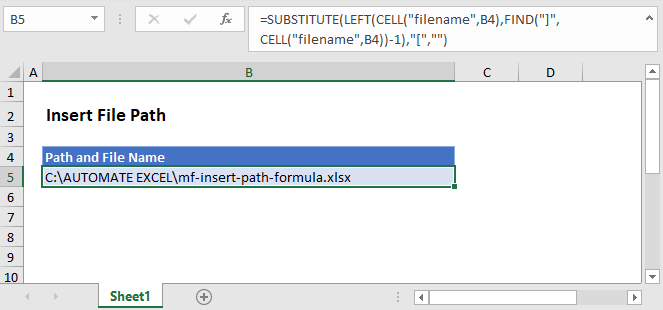## Get Path and File Name

In Excel there isn’t a function to get the path and file name directly, but the CELL Function will return the file path, name, and sheet. Using the text functions FIND, LEFT, and SUBSTITUTE, we can isolate the path and file name.

``=SUBSTITUTE(LEFT(CELL("filename",B2),FIND("]",CELL("filename",B2))-1),"[","")``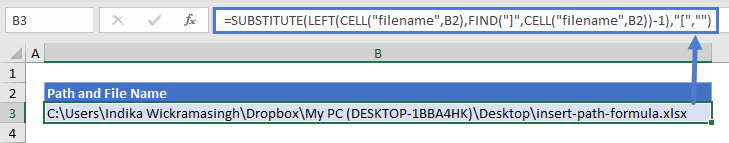Let’s step through the formula.

### File Name, Path, and Worksheet

We use the CELL Function to return the file path, name, and sheet by entering “filename” as the info type.

``=CELL(“filename”,B2)``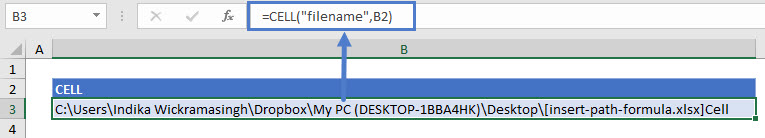### FIND the File Name Position

As shown above, the CELL Function returns the file path, name, and worksheet. We don’t need the worksheet or the square brackets, so we use the FIND function to determine the position of the last character (i.e. the one before “]”) of the file name.

``=FIND("]",B3)-1``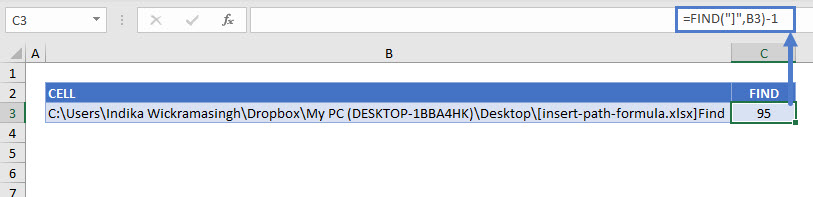### Remove the Worksheet Name

Once we have the position of the file name’s last character, we use the LEFT Function to remove the name of the worksheet.

``=LEFT(B3,C3)``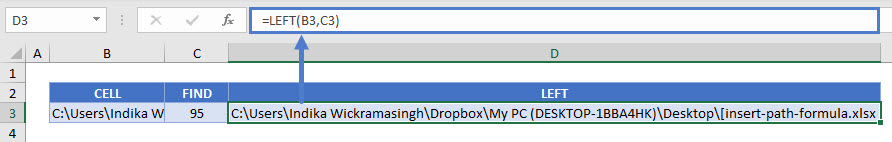### SUBSTITUTE Function

You can see above that there is still an open square bracket between the path and file names. Use the SUBSTITUTE function to replace the “[“ with an empty string.

``=SUBSTITUTE(D3,"[","")``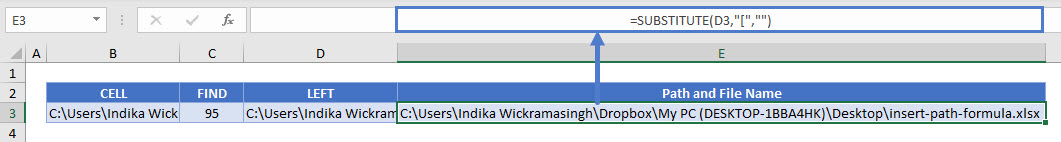Combining these steps into a single formula gives us:

``=SUBSTITUTE(LEFT(CELL("filename",B2),FIND("]",CELL("filename",B2))-1),"[","")``

## Get Path Only

You might want to show the path only, without the file name. For this, we can stop at the LEFT Function with a little tweak. There’s no need to SUBSTITUTE since there won’t be any mid-string characters to delete. To return just the path, we find the position of the first character of the file name (“[“), instead of the last, and the path name is everything to the left.

``=LEFT(CELL("filename",B2),FIND("[",CELL("filename",B2))-1)``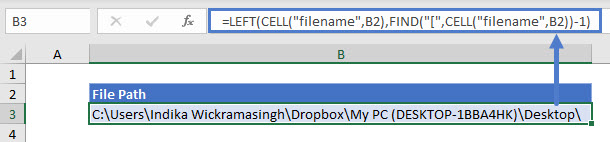### Excel Practice Worksheet

Practice Excel functions and formulas with our 100% free practice worksheets!